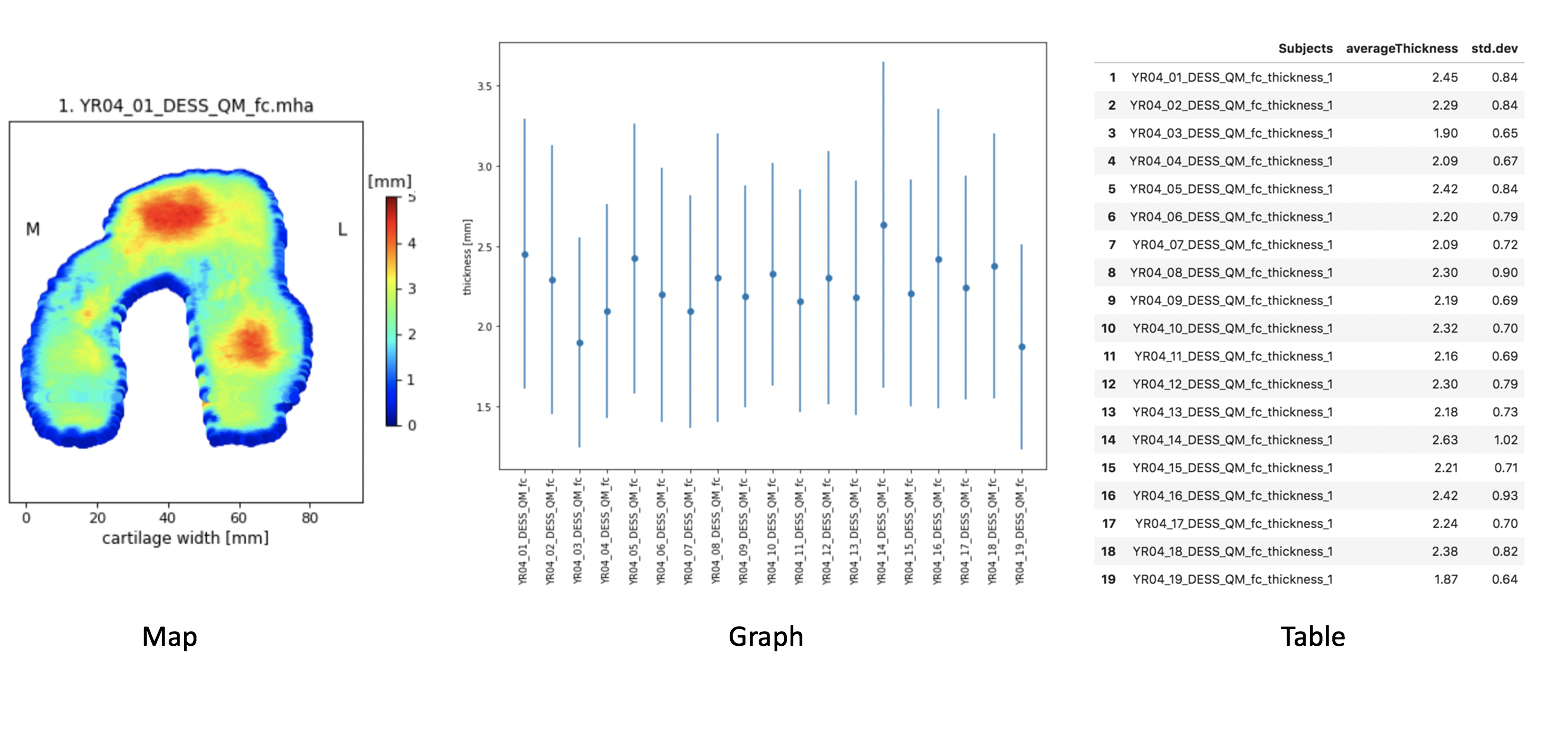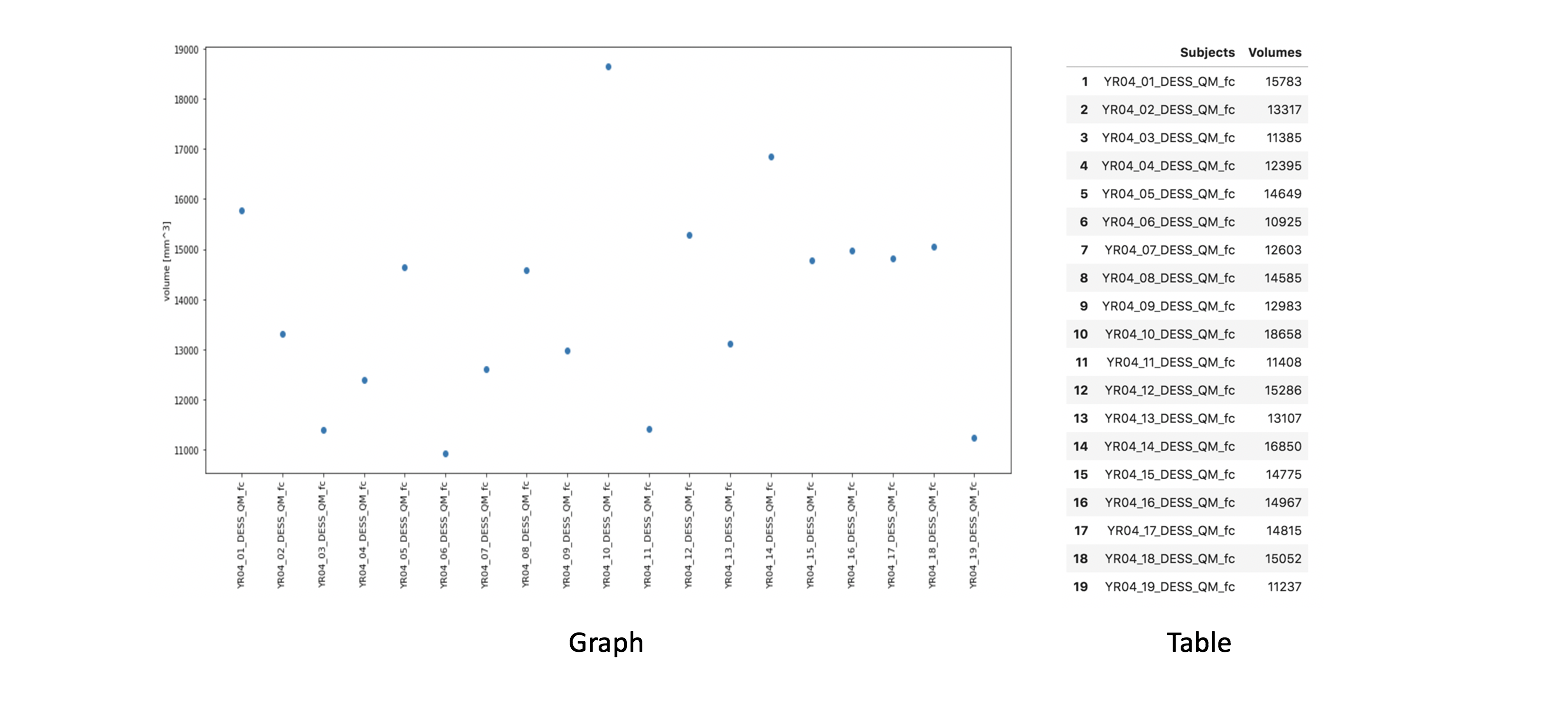# Morphology¶

pyKNEEr computes two quantitative metrics to characterize femoral cartilage morphology:

• Thickness, calculated in two steps:

• Separation of subcondral surface and articular surface of the cartilage

• Calculation of the distance between the two surfaces using a nearest neighbor method

• Volume, calculated as the volume of the cartilage mask multiplied by the voxel volume

Note

You can calculate cartilage thickness and volume independently although their computations are implemented in the same notebook

## Input: Image folder list¶

For the demo images, the input file is image_list_morphology.txt, which contains:

 ./segmented
 01_DESS_01_prep_fc.mha


where:

• Line 1: Input folder, containing the cartilage masks

• Line 2: Name of cartilage mask

Tip

• Customize image_list_morphology.txt with path and names of your own binary masks

## Executing morphology.ipynb¶

To compute femoral cartilage morphology:

• Launch Jupyter notebook

• In File Browser, navigate to morphology.ipynb, open it, and:

• Customize the input variables :

• Follow the instructions in the notebook {note} Cartilage thickness and volume are calculated independently, so it is not necessary to calculate thickness before calculating the volume

• Save your notebook at the end of the process

## Output: Surfaces, thicknesses, and volumes¶

The outputs are in the folder .morphology. For each subject, the outputs are:

• *_boneCart.txt: Subchondral surface of the cartilage as a points cloud. Each row corresponds to a point, and the three columns correspond to the x, y, and z coordinates

• *_artiCart.txt: Articular surface of the cartilage as a points cloud

• thickness_ID.txt: List of thicknesses in mm associated to the surface points

ID is the algorithm chosen to calculate cartilage thickness:

1. Nearest neighbor calculated on the femur surface

2. Nearest neighbor calculated on the articular surface

• volume.txt: Cartilage volume in $$mm^3$$

## Visualization: Map, graph, and table¶

• Cartilage thickness is visualized as:

• Map: Thickness values are a colormap on the cartilage surface, flattened after interpolation to a cylinder . The same flattening algorithm is used to visualize subcondral and articular cartilage after splitting

• Graph: Dots represent the average value of cartilage thickness per image and bars represent the standard deviation

• Table: Thickness average and standard deviation, also saved as .csv file for subsequent analysis• Cartilage volume is visualized as:

• Graph: Dots represent volume per image

• Table: Volume values, also saved as .csv file for subsequent analysis